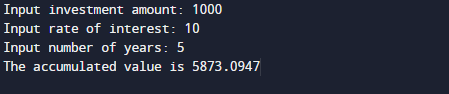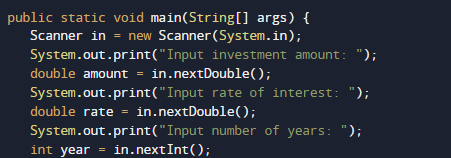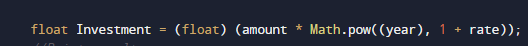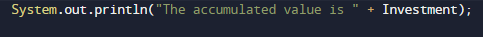# Java Program to Calculate Future Investment Value

In this tutorial you will learn about the Java Program to Calculate Future Investment Value and its application with practical example.

In this tutorial, we will learn to create a Java Program to Calculate Future Investment value in Java using Java programming.

## Prerequisites

Before starting with this tutorial we assume that you are best aware of the following Java programming topics:

• Java Operators.
• Basic Input and Output function in Java.
• Class and Object in Java.
• Basic Java programming.
• If-else statements in Java.
• For loop in Java.

## Investment

investing means buying an asset, or money put in a bank to get an interest in it in the future in other words investment is a process of allocating money in expectation of a benefit/return in the Future.

## Java Program to Calculate Future Investment Value

In our program, we will learn to calculate the Future investment value of a given Money. Firstly we declare the required variable and also initiate the required values in the variable. Next, we take value from the user at run time, and then after we will Calculate Future Investment value.

## Output

Investment.In the above program, we have first declared and initialized a set of variables required in the program.

• amount= it will hold the entered value of amount
• rate= it will hold the entered value of rate
• year= it will give the value of the year.
• Investment= it will give the value of the accumulated  Price.

First of all, we declared and initialized the set of variables required in the program. Then we will take a value from the user at run time as shown in the image below.After taking all the values for user we will calculate the future investment using the following formula.And finally with the help of above formula we will print the required estimation value of a invested amount.In this tutorial we have learn about the Java Program to Calculate Future Investment Value and its application with practical example. I hope you will like this tutorial.